Next: Covariance Estimation Up: Statistical Background Previous: Model Selecton

## Parameter Estimation

Often describing the cost function for an algorithm is not the only part of the algorithmic solution. We also need a way of searching a space of possible models in order to find one that optimizes this function. If we take the view that the parameters (or variables) in our system model correspond to unique, perhaps even physical, meaningful values then the algorithmic goal becomes that of parameter estimation (along with the associated covariances so that we can make practical use of the result).

There are many parameter estimation techniques, the commonest being those that seek to optimize a well behaved function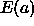subject to change in the model parameters a. A common approach here assumes that the functional form ofis approximately quadratic such that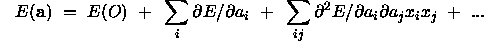This equation can be seen to be directly analogous to that used later in the discussion of covariance. For simplicity we will associate these terms with the expression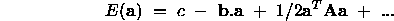if the quadratic approximation is accurate then clearly by differentiation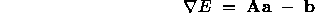which has a minimum when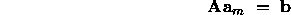thus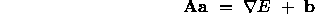therefore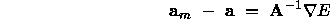This solution suggests an iterative update scheme for estimates of a close to a quadratic minimum and this idea forms the basis of `quasi-Newton' methods which provide iterative methods for approximating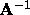. The popular Kalman filter is effectively an iterative form of such an algorithm which provides a mechanism for optimal combination of new data into the current estimate of the parameters.

These methods, and the associated `conjugate gradient' scheme require accurate derivative information which may not always be available. Under these circumstances methods which require only function evaluations such as the `downhill simplex' method may be used. This method can be recommended as a starting point for getting results quickly from a particular minimization problem. This method and the entire issue of function minimization is covered well in .

Sometimes the minimization function is not only badly behaved but so too is the parameter space a. Under these circumstances the whole concept of functional optimization needs to be reconsidered. At this point algorithmic research switches from an evaluation of the best optimization functions to designing new optimization methods. Such approaches are best typified by algorithms such as simulated annealing  and genetic algorithms . The main difference between these approaches is that the former operates on what are effectively single point trials of the optimization function while the latter operates with a set of interacting solutions. These interactions provide a very useful means for controlling the range of locations searched by the algorithm which is lacking from simulated annealing.

These algorithms can cope not only with multiple, discontinuous functions and parameter spaces but also non-stationary (even stochastic) evaluation functions. These properties are not however, obtained freely, the first obvious cost is the computation requirement. The success of simulated annealing is heavily influence by the choice of a problem specific annealing schedule. Genetic algorithms require that the parameters be cast in an appropriate representation (generally binary), suitable for the processes of cross-over and mutation which will drive the search efficiently through variants of the parameters in its search for the optimum. For these reasons these techniques are often looked upon as methods for solving specific long standing problems rather than automatic algorithmic solution to a class of problem.

The main point to be made on the process of function minimization is that the choice of minimization method (if adequate for the task) will not alter the performance of an overall algorithm. The main effect on algorithmic performance in the final evaluation lies with adequate definition of the cost function itself, which must have its roots in probability theory. If a set of data are insufficient to determine a set of parameters with sufficient accuracy for the task in hand then changing the method of optimization will not improve the situation.Next: Covariance Estimation Up: Statistical Background Previous: Model Selecton

Bob Fisher
Fri Mar 28 14:12:50 GMT 1997